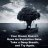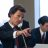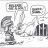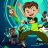# VX Futures Contango Indicator for ThinkorSwim

T

##### New member
Hi Hi, first time here.

Been reading through and have not yet found anything dealing with how to edit code to work after thinkorswim changed their futures symbol taxonomy. Code is posted below the tear-line. Can someone help a brother out? I've tried but cannot seem to make it work.

----------------------------------------
Code:
``````# Display Contango relationship between the Front and Back Month VX futures
#wizard text: Inputs: flavor:  0: commulative value, 1: relative commulative difference, 2: absolute difference
input bubles = 0;
input flavor = 3; # 0 for cumulative value, #1 for relative difference with respect to Front month, 2 for absolute difference.
# 3, for Contango % thresholds, from (BM-FM)*100/FM.
input Threshold_shortVol = 6.77;
input Threshold_longVol = 2.90;
declare lower;
#hidepriceplot(close);
#hide();
# Below flags the date for expiring Front Month VX futures per CBOE specs
# Note: Regarding the Holidays, only one occurrence since 2010, which is
# hard coded in line below.
# For some unknown reason, TOS does not respond to daily VX futures per
# specification before August 2010, so data seems valid after Aug 2010
# In debugging observed TOS sometimes misses
# complete trading days, the "/VX" reference in TOS does not
# match the CBOE spec for settlement and TOS "opportunities"
# seem to be dynamic (not static bugs)
# TOS inferred no trading of "/VX" between 7/16/2013 and 7/22/2016. (Missing 3 days of trading)
script VXexp {
def date = GetYYYYMMDD();
def isholiday = date == 20140319; # Manual injection of holiday on 4/18/14 which alters VX settlement date in March.
def n3rdfa = Next3rdFriday(1);
def n3rdfb = Next3rdFriday(2);
def n3rdf = if (n3rdfa < 4) then n3rdfb else n3rdfa; # Pick Next month's expiration, not this month.
plot VXexp = if (isholiday) then 1 else ((n3rdf == 30) and !isholiday);#and (dow == 3);
} # End of script for VXexp

# LsD extracts least significant digit of the number (date)
script LsD {
input date = 20100101;
plot LsD = (date - RoundDown(date / 10, 0) * 10);
} # End of script for LsD
def InContango;
def NotContango;
def ContangoBias;
def fm;
def bm;
def date = GetYYYYMMDD();
def dom = GetDayOfMonth(date);
def newmo = dom < dom;
def incmo = if newmo then 0 else if VXexp() then 1 else incmo;
def Y_ = GetYear();

def Y = LsD(Y_);
def MM = GetMonth();
fm = if (MM + incmo) > 12 then 1 else (MM + incmo) ;
def fmchange = fm != fm;
def fmY = if fmchange then (if (MM + incmo) > 12 then LsD(Y + 1) else Y) else fmY;
bm = if (fm + 1) > 12 then 1 else (fm + 1) ;
def bmY = if fmchange then (if (bm < 3) then LsD(Y + 1) else Y) else bmY;
def fmp = close( "/VX" + (if fm == 1 then "F" else
if fm == 2 then "G" else
if fm == 3 then "H" else
if fm == 4 then "J" else
if fm == 5 then "K" else
if fm == 6 then "M" else
if fm == 7 then "N" else
if fm == 8 then "Q" else
if fm == 9 then "U" else
if fm == 10 then "V" else
if fm == 11 then "X" else
if fm == 12 then "Z" else " ") + fmY);
def bmp = close( "/VX" + (if bm == 1 then "F" else
if bm == 2 then "G" else
if bm == 3 then "H" else
if bm == 4 then "J" else
if bm == 5 then "K" else
if bm == 6 then "M" else
if bm == 7 then "N" else
if bm == 8 then "Q" else
if bm == 9 then "U" else
if bm == 10 then "V" else
if bm == 11 then "X" else
if bm == 12 then "Z" else " ") + bmY);
# TOS may miss some vx futures quotes, so if this occurs
# re-use the prior day close for the missing entry.
# The filter below accomplishes this.
def fmpfiltered = if IsNaN(fmp) then  fmpfiltered  else fmp;
def bmpfiltered = if IsNaN(bmp) then  bmpfiltered  else bmp;
def reladj = if (bmpfiltered > 0) then bmpfiltered else 1;
#def fmpfiltered = if isnan(fmp) then if (fmpfiltered>0) then fmpfiltered else double.nan  else fmp;
#def bmpfiltered = if isnan(bmp) then if (bmpfiltered>0) then  bmpfiltered else double.nan else bmp;
def AbsDiffContango = if (BarNumber() < 5) then 0 else (if (flavor == 1) then (bmpfiltered - fmpfiltered) / reladj else (bmpfiltered - fmpfiltered));
ContangoBias = if (BarNumber() < 5) then 0 else ContangoBias + (if (flavor == 1) then (bmpfiltered - fmpfiltered) / reladj else (bmpfiltered - fmpfiltered));
plot FmBmContango = if IsNaN(fmp) then Double.NaN else if (flavor == 2) then
AbsDiffContango else if (flavor == 3) then Double.NaN else ContangoBias;
def ContangoPerCentage = 100 * AbsDiffContango / fmpfiltered;
FmBmContango.DefineColor("green", Color.GREEN);
FmBmContango.DefineColor("red", Color.RED);
FmBmContango.DefineColor("white", Color.WHITE);

plot Cper = if ((flavor == 3) and  (IsNaN(fmp) == 0)) then ContangoPerCentage else Double.NaN;
Cper.DefineColor("green", Color.GREEN);
Cper.DefineColor("red", Color.RED);
Cper.DefineColor("white", Color.WHITE);
Cper.AssignValueColor(if (ContangoPerCentage > Threshold_shortVol) then Cper.Color("green") else if (ContangoPerCentage > Threshold_longVol) then Cper.Color("white") else Cper.Color("red"));
Cper.SetPaintingStrategy(PaintingStrategy.POINTS);
def Direction = ContangoBias > ContangoBias;
def DirectionChanges = if (BarNumber() == 1) then 0 else if (Direction != Direction) then DirectionChanges + 1 else DirectionChanges;
FmBmContango.AssignValueColor(if (ContangoBias > ContangoBias) then FmBmContango.Color("green") else if (ContangoBias == ContangoBias) then FmBmContango.Color("white") else FmBmContango.Color("red"));
FmBmContango.SetPaintingStrategy(PaintingStrategy.POINTS);
InContango = if ((BarNumber() == 1) or (DirectionChanges < 1)) then 0 else InContango + (bmpfiltered > fmpfiltered);
NotContango = if ((BarNumber() == 1) or (DirectionChanges < 1)) then 0 else NotContango + (bmpfiltered <= fmpfiltered);

plot UpperThreshold = if (flavor == 3) then Threshold_shortVol else Double.NaN;
plot LowerThreshold = if (flavor == 3) then Threshold_longVol else Double.NaN;

AddChartBubble(bubles and Direction and !Direction, ContangoBias, DirectionChanges, Color.GRAY, 1);
AddChartBubble(bubles and !Direction and Direction, ContangoBias, DirectionChanges, Color.YELLOW, 0);

AddVerticalLine(VXexp(), "VX" + (if bm == 1 then "F" else
if bm == 2 then "G" else
if bm == 3 then "H" else
if bm == 4 then "J" else
if bm == 5 then "K" else
if bm == 6 then "M" else
if bm == 7 then "N" else
if bm == 8 then "Q" else
if bm == 9 then "U" else
if bm == 10 then "V" else
if bm == 11 then "X" else
if bm == 12 then "Z" else " ") + bmY
+ " - VX" + (if fm == 1 then "F" else
if fm == 2 then "G" else
if fm == 3 then "H" else
if fm == 4 then "J" else
if fm == 5 then "K" else
if fm == 6 then "M" else
if fm == 7 then "N" else
if fm == 8 then "Q" else
if fm == 9 then "U" else
if fm == 10 then "V" else
if fm == 11 then "X" else
if fm == 12 then "Z" else " ") + fmY, Color.DARK_GREEN);#color.light_orange);``````

Last edited by a moderator:
T

#### tomsk

##### Well-known member
VIP
Warehouse
I took a look at this over the thanksgiving holiday weekend.

About 2-3 years ago TOS modified the futures symbols to append extensions like XCME, XCBF, XNYM
Before TOS made these modifications, programs such as that you've posted worked just fine.
Unfortunately there are no string manipulation capabilities on TOS either so that complicates things
Hence there is no easy answer.

Load the following one liner study on any futures symbols like /ES, /VX, /GC, etc and you'll see what I mean
Now try loading charts like AAPL, FB, NFLX, etc - they don't have any extraneous symbols tacked on to them

AddLabel(1, "Symbol = " + getSymbol(), Color.Yellow);

Last edited:
•T

Thanks tomsk

T

#### tomsk

##### Well-known member
VIP
Warehouse
Thanks tomsk
You're very welcome! It's a real shame as that study was real nice when it worked way back in 2015/2016
I keep track of backwardation events on a weekly basis just by monitoring the front month and back month /VX futures
Currently those contracts are /VXZ19 and /VXF20, we are less than 2 points to a backwardation state
Hope this helps.

T

#### tomsk

##### Well-known member
VIP
Warehouse
Folks I had a bit of spare time this weekend and decided to rip the guts out of this legacy /VX futures contango study that @theloneytrader posted several weeks ago, to explore what possibilities we can do with this.

After looking at the underlying code logic, decided to do a major overhaul on how the values of the front/back month vol futures could be extracted from TOS. Note that the underlying logic of the contango calculations and relationships that the author engineered had been preserved. At least now we can see some data points being plotted.

Here then is the revamped and cleaned up overhaul code of the study. Make sure you run this on daily aggregations.

Code:
``````# VX Futures Contango
# tomsk
# 12.8.2019

# V1.0 - 11.28.2019 - thelonelytrader - Initial version of posted study
# V2.0 - 12.08.2019 - tomsk           - Major revamped version to handle /VX taxonomy

# Displays Contango relationship between the Front and Back Month /VX futures
#
# According to the design from the original author, there are three possible
# input flavors. It is set to default to option #3, for contango % thresholds
#
# 1 For relative cumulative difference with respect to Front month
# 2 For absolute difference.
# 3 For Contango % thresholds, from (BM-FM)*100/FM.

declare lower;

input showBubbles = no;
input showDebug = no;
input Threshold_shortVol = 6.77;
input Threshold_longVol = 2.90;
input flavor = 3;

# Below flags the date for expiring Front Month /VX futures per CBOE specs
# Note: Regarding the Holidays, only one occurrence since 2010, which is
# hard coded in line below. For some unknown reason, TOS does not respond
# to daily /VX futures per specification before August 2010, so data seems
# valid after Aug 2010
#
# In debugging observed TOS sometimes misses complete trading days, the
# "/VX" reference in TOS does not match the CBOE spec for settlement and
# TOS "opportunities" seem to be dynamic (not static bugs). TOS inferred no
# trading of "/VX" between 7/16/2013 and 7/22/2016. (Missing 3 trading days)

script VXexp {
def date = GetYYYYMMDD();
def isholiday = date == 20140319; # Manual injection of holiday on 4/18/14 which alters VX settlement date in March.
def n3rdfa = Next3rdFriday(1);
def n3rdfb = Next3rdFriday(2);
def n3rdf = if (n3rdfa < 4) then n3rdfb else n3rdfa; # Pick Next month's expiration, not this month.
plot VXexp = if (isholiday) then 1 else ((n3rdf == 30) and !isholiday);#and (dow == 3);
}

script LsD {
input date = 2010;
plot LsD = date - 2000;
}

def date = GetYYYYMMDD();
def dom = GetDayOfMonth(date);
def newmo = dom < dom;
def incmo = if newmo then 0 else if VXexp() then 1 else incmo;
def Y_ = GetYear();
def Y = LsD(Y_);
def MM = GetMonth();

def fm = if (MM + incmo) > 12 then 1 else (MM + incmo);
def fmchange = fm != fm;
def fmY = if fmchange then (if (MM + incmo) > 12 then LsD(Y + 1) else Y) else fmY;
def bm = if (fm + 1) > 12 then 1 else (fm + 1);
def bmY = if fmchange then (if (bm < 3) then LsD(Y_ + 1) else Y) else bmY;

AddLabel(showDebug, "Back Month Symbol = " + ((if bm == 1  then "/VXF" else
if bm == 2  then "/VXG" else
if bm == 3  then "/VXH" else
if bm == 4  then "/VXJ" else
if bm == 5  then "/VXK" else
if bm == 6  then "/VXM" else
if bm == 7  then "/VXN" else
if bm == 8  then "/VXQ" else
if bm == 9  then "/VXU" else
if bm == 10 then "/VXV" else
if bm == 11 then "/VXX" else
if bm == 12 then "/VXZ" else " ") + bmY), Color.PINK);
def fmp = close(symbol = "/VX");
def bmp = close((if bm == 1  then "/VXF" else
if bm == 2  then "/VXG" else
if bm == 3  then "/VXH" else
if bm == 4  then "/VXJ" else
if bm == 5  then "/VXK" else
if bm == 6  then "/VXM" else
if bm == 7  then "/VXN" else
if bm == 8  then "/VXQ" else
if bm == 9  then "/VXU" else
if bm == 10 then "/VXV" else
if bm == 11 then "/VXX" else
if bm == 12 then "/VXZ" else " ") + bmY);

AddLabel(showDebug, "fmp = " + fmp, Color.YELLOW);
AddLabel(showDebug, "bmp = " + bmp, Color.YELLOW);

# TOS may miss some vx futures quotes, so if this occurs re-use the prior
# day close for the missing entry. The filter below accomplishes this.

def fmpfiltered = if IsNaN(fmp) then  fmpfiltered  else fmp;
def bmpfiltered = if IsNaN(bmp) then  bmpfiltered  else bmp;
def reladj = if (bmpfiltered > 0) then bmpfiltered else 1;

# def fmpfiltered = if isnan(fmp) then if (fmpfiltered>0) then fmpfiltered else double.nan  else fmp;
# def bmpfiltered = if isnan(bmp) then if (bmpfiltered>0) then  bmpfiltered else double.nan else bmp;

def AbsDiffContango = if (BarNumber() < 5)
then 0
else (if (flavor == 1)
then (bmpfiltered - fmpfiltered) / reladj
else (bmpfiltered - fmpfiltered));
def ContangoBias = if (BarNumber() < 5)
then 0
else ContangoBias + (if (flavor == 1)
then (bmpfiltered - fmpfiltered) / reladj
else (bmpfiltered - fmpfiltered));
plot FmBmContango = if IsNaN(fmp) then Double.NaN
else if (flavor == 2)
then AbsDiffContango
else if (flavor == 3)
then Double.NaN
else ContangoBias;
FmBmContango.DefineColor("green", Color.GREEN);
FmBmContango.DefineColor("red", Color.RED);
FmBmContango.DefineColor("white", Color.WHITE);

def ContangoPerCentage = 100 * AbsDiffContango / fmpfiltered;

plot Cper = if ((flavor == 3) and  (IsNaN(fmp) == 0)) then ContangoPerCentage else Double.NaN;
Cper.DefineColor("Green", Color.GREEN);
Cper.DefineColor("Red", Color.RED);
Cper.DefineColor("White", Color.WHITE);
Cper.AssignValueColor(if (ContangoPerCentage > Threshold_shortVol) then Cper.Color("Green")
else if (ContangoPerCentage > Threshold_longVol) then Cper.Color("White")
else Cper.Color("Red"));
Cper.SetPaintingStrategy(PaintingStrategy.POINTS);

def Direction = ContangoBias > ContangoBias;
def DirectionChanges = if (BarNumber() == 1) then 0
else if (Direction != Direction) then DirectionChanges + 1
else DirectionChanges;
FmBmContango.AssignValueColor(if (ContangoBias > ContangoBias) then FmBmContango.Color("Green")
else if (ContangoBias == ContangoBias) then FmBmContango.Color("White")
else FmBmContango.Color("Red"));
FmBmContango.SetPaintingStrategy(PaintingStrategy.POINTS);

def InContango = if ((BarNumber() == 1) or (DirectionChanges < 1)) then 0 else InContango + (bmpfiltered > fmpfiltered);
def NotContango = if ((BarNumber() == 1) or (DirectionChanges < 1)) then 0 else NotContango + (bmpfiltered <= fmpfiltered);

plot UpperThreshold = if (flavor == 3) then Threshold_shortVol else Double.NaN;
plot LowerThreshold = if (flavor == 3) then Threshold_longVol else Double.NaN;

AddChartBubble(showBubbles and Direction and !Direction, ContangoBias, DirectionChanges, Color.GRAY, 1);
AddChartBubble(showBubbles and !Direction and Direction, ContangoBias, DirectionChanges, Color.YELLOW, 0);
•Questions 3Questions 2Index Futures 5Index Futures 1